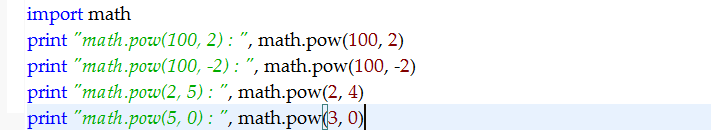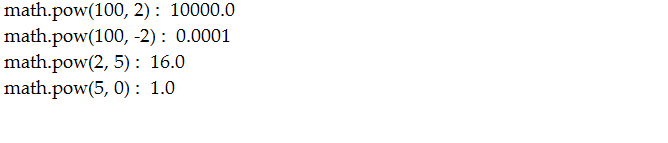在看一些關於關於資料分析的Python程式碼時，時常會出現一些方法不懂其意思，今天做個小小的說明關於:

python中numpy.clip（）方法的運用、numpy.fabs()方法運用法以及math.pow()的運用
<1>numpy.clip(a,a_min,a_max,a=None)的運用：

numpy.clip(a,a_min,a_max,a=None)方法的舉例運用。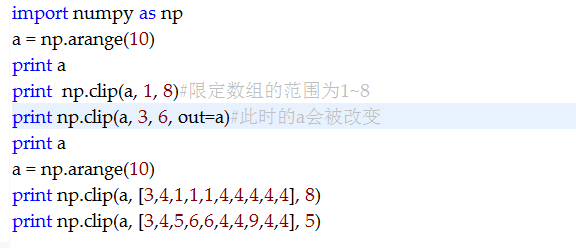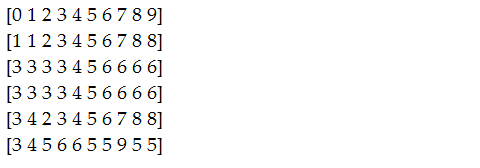<2>numpy.fabs(x)方法的運用：

numpy.fabs(x)方法的舉例運用：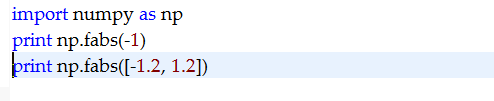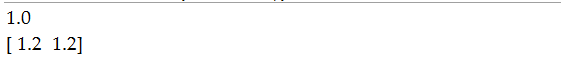<3>math.pow(x,y)的說明：

math.pow(x,y)的舉例說明：